Maths-
General
Easy

Question

# A hemispherical tank is made up of an iron sheet 1 cm thick. If the inner radius is 1m , then find the volume of the iron used to make the tank ?Hint:

## The correct answer is: 0.06348m3

### Explanation: We have given a hemisphere with thickness 1cm. We have to find the volume of iron used to make the tank. Step 1 of 1:Since the hemispherical tank is made of 1cm thick iron, we can find the outer radius of the tank by adding thickness to the inner radius.The Volume of hemisphere of base radius r is equal to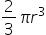The inner radius of the tank r = 1mThickness of iron = 1cm = 1/100 m =0.01mOuter radius of the tank, R = 1m + 0.01m = 1.01mThe volume of the iron used to make the tank can be calculated by subtracting the volume of the tank with inner radius from the volume of the tank with outer radius.Volume of the iron used to make the tank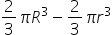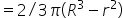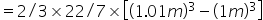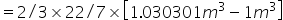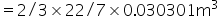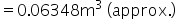0.06348 m3 of iron used to make the tank#### With Turito Foundation.#### Get an Expert Advice From Turito.